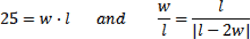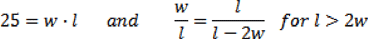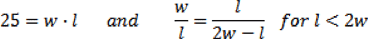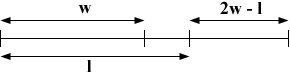SEARCH HOMEMath Central Quandaries & QueriesQuestion from Charlene, a student: The ratio of the width to the length of a certain rectangle is equal to the ratio of its length to the difference between its length and twice its width. If the area of the rectangle is 25cm^2, then what are its dimensions? Present a non- algebraic solution?Hello,
Let  w  be the width, and  l  be the length of the rectangle.
The information given amounts to stating that:Where |l - 2w|  is the absolute value of the difference between the length and twice the width.

(Both  w  and  l  are positive numbers, so it doesn't make sense for (l - 2w) to be negative if the second equation is to be satisfied)
Therefore we have two cases:
Case 1   Solve for  w  and  l  given:Case 2   Solve for  w  and  l  given:You will find that one case is not possible.

For a non-algebraic solution, consider a line segment divided up as such:The length of   w  is to  l  as length of  l  is to  2w – l ?
How long does  2w – l  have to be for our equation to make sense?

Hope this helps,
TylerMath Central is supported by the University of Regina and The Pacific Institute for the Mathematical Sciences.